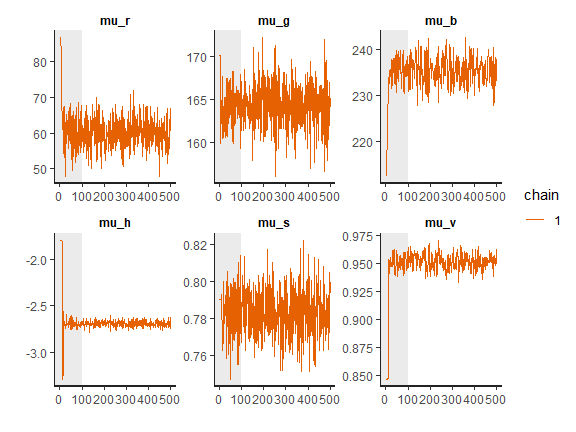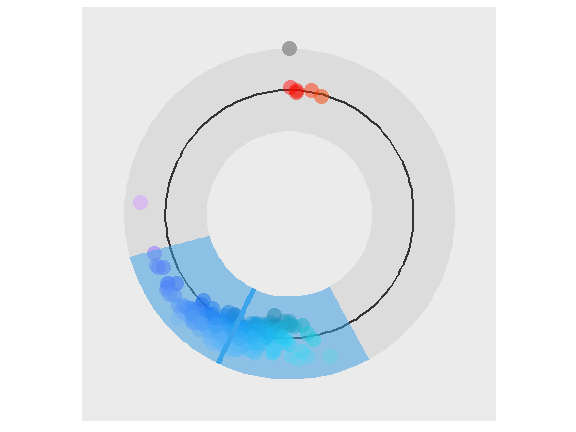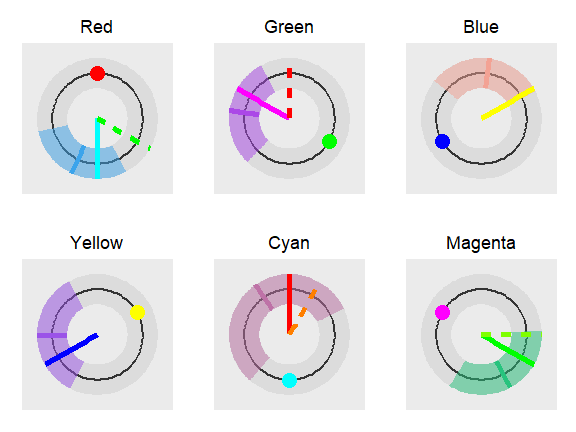# Introduction

In the afterimages task participants were asked to fix their gaze on a fixation point in the middle of the computer screen. Stimulus – a colored rectangle – was then shown above the fixation point. After 20 seconds the rectangle disappeared and a color palette was shown on the right-hand side of the screen. Participants were asked to keep their gaze on the fixation point while using the mouse to select the color that best matches the color of the afterimage that appeared above the fixation point. Then a colored rectangle of the selected color and same size as before was shown below the fixation point. Finally, participants confirmed their selection. For each trial the color of the stimulus rectangle, the response in RGB and the response time were recorded. The goal of this study was to determine which of the two color coding mechanisms (trichromatic or opponent-process), better explains the color of the afterimages. We used six differently colored rectangles: red, green, blue, cyan, magenta, yellow.

We start our analysis by loading the experiment and stimuli data. The experiment data include subject index, reaction time, response in RGB format, stimuli name (e.g blue) and stimuli values in RGB and HSV. The stimuli data set includes only the information about stimuli (names, RGB and HSV values).

# libs
library(bayes4psy)
library(cowplot)
library(dplyr)
library(ggplot2)

data_all <- after_images

stimuli <- after_images_stimuli

Once we load required libraries and thata we can start fitting the Bayesian color models. Below is a detailed example of fitting the Bayesian color model for red color stimuli. Note here that, due to vignette limitations, all fits are built using only one chain, using more chains in parallel is usually more efficient. Also to increase the building speed of vignettes we greatly reduced the amount of iterations, use an appropriate amount of iterations when executing actual analyses!

# prepare data
data_red <- data_all %>% filter(stimuli == "red")
data_red <- data.frame(r=data_red$r, g=data_red$g,
b=data_red$b) # fit fit_red <- b_color(colors=data_red, chains=1, iter=500, warmup=100) ## ## SAMPLING FOR MODEL 'color' NOW (CHAIN 1). ## Chain 1: ## Chain 1: Gradient evaluation took 0.001 seconds ## Chain 1: 1000 transitions using 10 leapfrog steps per transition would take 10 seconds. ## Chain 1: Adjust your expectations accordingly! ## Chain 1: ## Chain 1: ## Chain 1: WARNING: There aren't enough warmup iterations to fit the ## Chain 1: three stages of adaptation as currently configured. ## Chain 1: Reducing each adaptation stage to 15%/75%/10% of ## Chain 1: the given number of warmup iterations: ## Chain 1: init_buffer = 14 ## Chain 1: adapt_window = 76 ## Chain 1: term_buffer = 10 ## Chain 1: ## Chain 1: Iteration: 1 / 500 [ 0%] (Warmup) ## Chain 1: Iteration: 50 / 500 [ 10%] (Warmup) ## Chain 1: Iteration: 100 / 500 [ 20%] (Warmup) ## Chain 1: Iteration: 101 / 500 [ 20%] (Sampling) ## Chain 1: Iteration: 150 / 500 [ 30%] (Sampling) ## Chain 1: Iteration: 200 / 500 [ 40%] (Sampling) ## Chain 1: Iteration: 250 / 500 [ 50%] (Sampling) ## Chain 1: Iteration: 300 / 500 [ 60%] (Sampling) ## Chain 1: Iteration: 350 / 500 [ 70%] (Sampling) ## Chain 1: Iteration: 400 / 500 [ 80%] (Sampling) ## Chain 1: Iteration: 450 / 500 [ 90%] (Sampling) ## Chain 1: Iteration: 500 / 500 [100%] (Sampling) ## Chain 1: ## Chain 1: Elapsed Time: 0.396 seconds (Warm-up) ## Chain 1: 1.457 seconds (Sampling) ## Chain 1: 1.853 seconds (Total) ## Chain 1: # inspect plot_trace(fit_red)print(fit_red) ## Inference for Stan model: color. ## 1 chains, each with iter=500; warmup=100; thin=1; ## post-warmup draws per chain=400, total post-warmup draws=400. ## ## mean se_mean sd 2.5% 25% 50% 75% 97.5% n_eff Rhat ## mu_r 60.08 0.21 4.00 52.60 57.32 60.10 62.85 67.70 354 1.00 ## sigma_r 58.11 0.08 2.57 53.41 56.45 57.85 59.58 63.55 1041 1.00 ## mu_g 164.26 0.11 2.69 158.66 162.58 164.14 166.09 169.53 573 1.01 ## sigma_g 44.41 0.08 1.96 40.52 43.13 44.28 45.59 48.60 640 1.00 ## mu_b 235.46 0.21 2.85 230.20 233.47 235.52 237.47 240.64 189 1.00 ## sigma_b 45.05 0.07 2.14 41.37 43.48 44.83 46.68 49.12 892 1.00 ## mu_h -2.69 0.00 0.03 -2.76 -2.71 -2.70 -2.67 -2.64 389 1.00 ## kappa_h 5.22 0.02 0.48 4.37 4.86 5.19 5.54 6.15 425 1.00 ## mu_s 0.78 0.00 0.01 0.76 0.77 0.78 0.79 0.81 536 1.00 ## sigma_s 0.21 0.00 0.01 0.19 0.20 0.21 0.21 0.23 855 1.00 ## mu_v 0.95 0.00 0.01 0.94 0.95 0.95 0.96 0.96 133 1.00 ## sigma_v 0.10 0.00 0.01 0.09 0.10 0.10 0.11 0.12 1041 1.00 ## lp__ -1940.36 0.16 2.32 -1945.51 -1941.83 -1940.09 -1938.59 -1936.84 211 1.01 ## ## Samples were drawn using NUTS(diag_e) at Wed Feb 19 11:04:25 2020. ## For each parameter, n_eff is a crude measure of effective sample size, ## and Rhat is the potential scale reduction factor on split chains (at ## convergence, Rhat=1). plot_hsv(fit_red)The function plot_hsv was developed specially for the color model. Input data points are visualized with circles, mean of the fit is visualized with a solid line and the 95% HDI of the underlying distribution is visualized as a colored band. If we are satisfied with the fit we repeat the whole process five more times for the remaining five colors of stimuli. For brevity only code for fitting the model is executed here, the inspections of fits are ommited. # green data_green <- data_all %>% filter(stimuli == "green") data_green <- data.frame(r=data_green$r,
g=data_green$g, b=data_green$b)
fit_green <- b_color(colors=data_green, chains=1, iter=500, warmup=100)
##
## SAMPLING FOR MODEL 'color' NOW (CHAIN 1).
## Chain 1:
## Chain 1: Gradient evaluation took 0 seconds
## Chain 1: 1000 transitions using 10 leapfrog steps per transition would take 0 seconds.
## Chain 1:
## Chain 1:
## Chain 1: WARNING: There aren't enough warmup iterations to fit the
## Chain 1:          three stages of adaptation as currently configured.
## Chain 1:          Reducing each adaptation stage to 15%/75%/10% of
## Chain 1:          the given number of warmup iterations:
## Chain 1:            init_buffer = 14
## Chain 1:            adapt_window = 76
## Chain 1:            term_buffer = 10
## Chain 1:
## Chain 1: Iteration:   1 / 500 [  0%]  (Warmup)
## Chain 1: Iteration:  50 / 500 [ 10%]  (Warmup)
## Chain 1: Iteration: 100 / 500 [ 20%]  (Warmup)
## Chain 1: Iteration: 101 / 500 [ 20%]  (Sampling)
## Chain 1: Iteration: 150 / 500 [ 30%]  (Sampling)
## Chain 1: Iteration: 200 / 500 [ 40%]  (Sampling)
## Chain 1: Iteration: 250 / 500 [ 50%]  (Sampling)
## Chain 1: Iteration: 300 / 500 [ 60%]  (Sampling)
## Chain 1: Iteration: 350 / 500 [ 70%]  (Sampling)
## Chain 1: Iteration: 400 / 500 [ 80%]  (Sampling)
## Chain 1: Iteration: 450 / 500 [ 90%]  (Sampling)
## Chain 1: Iteration: 500 / 500 [100%]  (Sampling)
## Chain 1:
## Chain 1:  Elapsed Time: 0.372 seconds (Warm-up)
## Chain 1:                1.364 seconds (Sampling)
## Chain 1:                1.736 seconds (Total)
## Chain 1:
# blue
data_blue <- data_all %>% filter(stimuli == "blue")
data_blue <- data.frame(r=data_blue$r, g=data_blue$g,
b=data_blue$b) fit_blue <- b_color(colors=data_blue, chains=1, iter=500, warmup=100) ## ## SAMPLING FOR MODEL 'color' NOW (CHAIN 1). ## Chain 1: ## Chain 1: Gradient evaluation took 0 seconds ## Chain 1: 1000 transitions using 10 leapfrog steps per transition would take 0 seconds. ## Chain 1: Adjust your expectations accordingly! ## Chain 1: ## Chain 1: ## Chain 1: WARNING: There aren't enough warmup iterations to fit the ## Chain 1: three stages of adaptation as currently configured. ## Chain 1: Reducing each adaptation stage to 15%/75%/10% of ## Chain 1: the given number of warmup iterations: ## Chain 1: init_buffer = 14 ## Chain 1: adapt_window = 76 ## Chain 1: term_buffer = 10 ## Chain 1: ## Chain 1: Iteration: 1 / 500 [ 0%] (Warmup) ## Chain 1: Iteration: 50 / 500 [ 10%] (Warmup) ## Chain 1: Iteration: 100 / 500 [ 20%] (Warmup) ## Chain 1: Iteration: 101 / 500 [ 20%] (Sampling) ## Chain 1: Iteration: 150 / 500 [ 30%] (Sampling) ## Chain 1: Iteration: 200 / 500 [ 40%] (Sampling) ## Chain 1: Iteration: 250 / 500 [ 50%] (Sampling) ## Chain 1: Iteration: 300 / 500 [ 60%] (Sampling) ## Chain 1: Iteration: 350 / 500 [ 70%] (Sampling) ## Chain 1: Iteration: 400 / 500 [ 80%] (Sampling) ## Chain 1: Iteration: 450 / 500 [ 90%] (Sampling) ## Chain 1: Iteration: 500 / 500 [100%] (Sampling) ## Chain 1: ## Chain 1: Elapsed Time: 0.395 seconds (Warm-up) ## Chain 1: 1.945 seconds (Sampling) ## Chain 1: 2.34 seconds (Total) ## Chain 1: # yellow data_yellow <- data_all %>% filter(stimuli == "yellow") data_yellow <- data.frame(r=data_yellow$r,
g=data_yellow$g, b=data_yellow$b)
fit_yellow <- b_color(colors=data_yellow, chains=1, iter=500, warmup=100)
##
## SAMPLING FOR MODEL 'color' NOW (CHAIN 1).
## Chain 1:
## Chain 1: Gradient evaluation took 0 seconds
## Chain 1: 1000 transitions using 10 leapfrog steps per transition would take 0 seconds.
## Chain 1:
## Chain 1:
## Chain 1: WARNING: There aren't enough warmup iterations to fit the
## Chain 1:          three stages of adaptation as currently configured.
## Chain 1:          Reducing each adaptation stage to 15%/75%/10% of
## Chain 1:          the given number of warmup iterations:
## Chain 1:            init_buffer = 14
## Chain 1:            adapt_window = 76
## Chain 1:            term_buffer = 10
## Chain 1:
## Chain 1: Iteration:   1 / 500 [  0%]  (Warmup)
## Chain 1: Iteration:  50 / 500 [ 10%]  (Warmup)
## Chain 1: Iteration: 100 / 500 [ 20%]  (Warmup)
## Chain 1: Iteration: 101 / 500 [ 20%]  (Sampling)
## Chain 1: Iteration: 150 / 500 [ 30%]  (Sampling)
## Chain 1: Iteration: 200 / 500 [ 40%]  (Sampling)
## Chain 1: Iteration: 250 / 500 [ 50%]  (Sampling)
## Chain 1: Iteration: 300 / 500 [ 60%]  (Sampling)
## Chain 1: Iteration: 350 / 500 [ 70%]  (Sampling)
## Chain 1: Iteration: 400 / 500 [ 80%]  (Sampling)
## Chain 1: Iteration: 450 / 500 [ 90%]  (Sampling)
## Chain 1: Iteration: 500 / 500 [100%]  (Sampling)
## Chain 1:
## Chain 1:  Elapsed Time: 0.358 seconds (Warm-up)
## Chain 1:                1.706 seconds (Sampling)
## Chain 1:                2.064 seconds (Total)
## Chain 1:
# cyan
data_cyan <- data_all %>% filter(stimuli == "cyan")
data_cyan <- data.frame(r=data_cyan$r, g=data_cyan$g,
b=data_cyan$b) fit_cyan <- b_color(colors=data_cyan, chains=1, iter=500, warmup=100) ## ## SAMPLING FOR MODEL 'color' NOW (CHAIN 1). ## Chain 1: ## Chain 1: Gradient evaluation took 0 seconds ## Chain 1: 1000 transitions using 10 leapfrog steps per transition would take 0 seconds. ## Chain 1: Adjust your expectations accordingly! ## Chain 1: ## Chain 1: ## Chain 1: WARNING: There aren't enough warmup iterations to fit the ## Chain 1: three stages of adaptation as currently configured. ## Chain 1: Reducing each adaptation stage to 15%/75%/10% of ## Chain 1: the given number of warmup iterations: ## Chain 1: init_buffer = 14 ## Chain 1: adapt_window = 76 ## Chain 1: term_buffer = 10 ## Chain 1: ## Chain 1: Iteration: 1 / 500 [ 0%] (Warmup) ## Chain 1: Iteration: 50 / 500 [ 10%] (Warmup) ## Chain 1: Iteration: 100 / 500 [ 20%] (Warmup) ## Chain 1: Iteration: 101 / 500 [ 20%] (Sampling) ## Chain 1: Iteration: 150 / 500 [ 30%] (Sampling) ## Chain 1: Iteration: 200 / 500 [ 40%] (Sampling) ## Chain 1: Iteration: 250 / 500 [ 50%] (Sampling) ## Chain 1: Iteration: 300 / 500 [ 60%] (Sampling) ## Chain 1: Iteration: 350 / 500 [ 70%] (Sampling) ## Chain 1: Iteration: 400 / 500 [ 80%] (Sampling) ## Chain 1: Iteration: 450 / 500 [ 90%] (Sampling) ## Chain 1: Iteration: 500 / 500 [100%] (Sampling) ## Chain 1: ## Chain 1: Elapsed Time: 0.243 seconds (Warm-up) ## Chain 1: 1.336 seconds (Sampling) ## Chain 1: 1.579 seconds (Total) ## Chain 1: # magenta data_magenta <- data_all %>% filter(stimuli == "magenta") data_magenta <- data.frame(r=data_magenta$r,
g=data_magenta$g, b=data_magenta$b)
fit_magenta <- b_color(colors=data_magenta, chains=1, iter=500, warmup=100)
##
## SAMPLING FOR MODEL 'color' NOW (CHAIN 1).
## Chain 1:
## Chain 1: Gradient evaluation took 0 seconds
## Chain 1: 1000 transitions using 10 leapfrog steps per transition would take 0 seconds.
## Chain 1:
## Chain 1:
## Chain 1: WARNING: There aren't enough warmup iterations to fit the
## Chain 1:          three stages of adaptation as currently configured.
## Chain 1:          Reducing each adaptation stage to 15%/75%/10% of
## Chain 1:          the given number of warmup iterations:
## Chain 1:            init_buffer = 14
## Chain 1:            adapt_window = 76
## Chain 1:            term_buffer = 10
## Chain 1:
## Chain 1: Iteration:   1 / 500 [  0%]  (Warmup)
## Chain 1: Iteration:  50 / 500 [ 10%]  (Warmup)
## Chain 1: Iteration: 100 / 500 [ 20%]  (Warmup)
## Chain 1: Iteration: 101 / 500 [ 20%]  (Sampling)
## Chain 1: Iteration: 150 / 500 [ 30%]  (Sampling)
## Chain 1: Iteration: 200 / 500 [ 40%]  (Sampling)
## Chain 1: Iteration: 250 / 500 [ 50%]  (Sampling)
## Chain 1: Iteration: 300 / 500 [ 60%]  (Sampling)
## Chain 1: Iteration: 350 / 500 [ 70%]  (Sampling)
## Chain 1: Iteration: 400 / 500 [ 80%]  (Sampling)
## Chain 1: Iteration: 450 / 500 [ 90%]  (Sampling)
## Chain 1: Iteration: 500 / 500 [100%]  (Sampling)
## Chain 1:
## Chain 1:  Elapsed Time: 0.394 seconds (Warm-up)
## Chain 1:                1.677 seconds (Sampling)
## Chain 1:                2.071 seconds (Total)
## Chain 1:

We start the analysis by loading data about the colors predicted by the trichromatic or the opponent-process theory. We can then use the plot_distributions_hsv function of the Bayesian color model to produce a visualization of the accuracy of both color coding mechanisms predictions for each stimuli independently. Each graph visualizes the fitted distribution, displayed stimuli and responses predicted by the trichromatic and opponent-process coding. This additional information can be added to the visualization via annotation points and lines.

# load theory predictions
trichromatic <- after_images_trichromatic
opponent_process <- after_images_opponent_process

# red
stimulus <- "red"
lines <- list()
lines[] <- c(trichromatic[trichromatic$stimuli == stimulus, ]$h,
trichromatic[trichromatic$stimuli == stimulus, ]$s,
trichromatic[trichromatic$stimuli == stimulus, ]$v)
lines[] <- c(opponent_process[opponent_process$stimuli == stimulus, ]$h,
opponent_process[opponent_process$stimuli == stimulus, ]$s,
opponent_process[opponent_process$stimuli == stimulus, ]$v)

points <- list()
points[] <- c(stimuli[stimuli$stimuli == stimulus, ]$h_s,
stimuli[stimuli$stimuli == stimulus, ]$s_s,
stimuli[stimuli$stimuli == stimulus, ]$v_s)

plot_red <- plot_distributions_hsv(fit_red, points=points,
lines=lines, hsv=TRUE)

plot_red <- plot_red + ggtitle("Red") +
theme(plot.title = element_text(hjust = 0.5))

# green
stimulus <- "green"
lines <- list()
lines[] <- c(trichromatic[trichromatic$stimuli == stimulus, ]$h,
trichromatic[trichromatic$stimuli == stimulus, ]$s,
trichromatic[trichromatic$stimuli == stimulus, ]$v)
lines[] <- c(opponent_process[opponent_process$stimuli == stimulus, ]$h,
opponent_process[opponent_process$stimuli == stimulus, ]$s,
opponent_process[opponent_process$stimuli == stimulus, ]$v)

points <- list()
points[] <- c(stimuli[stimuli$stimuli == stimulus, ]$h_s,
stimuli[stimuli$stimuli == stimulus, ]$s_s,
stimuli[stimuli$stimuli == stimulus, ]$v_s)

plot_green <- plot_distributions_hsv(fit_green, points=points,
lines=lines, hsv=TRUE)
plot_green <- plot_green + ggtitle("Green") +
theme(plot.title = element_text(hjust = 0.5))

# blue
stimulus <- "blue"
lines <- list()
lines[] <- c(trichromatic[trichromatic$stimuli == stimulus, ]$h,
trichromatic[trichromatic$stimuli == stimulus, ]$s,
trichromatic[trichromatic$stimuli == stimulus, ]$v)
lines[] <- c(opponent_process[opponent_process$stimuli == stimulus, ]$h,
opponent_process[opponent_process$stimuli == stimulus, ]$s,
opponent_process[opponent_process$stimuli == stimulus, ]$v)

points <- list()
points[] <- c(stimuli[stimuli$stimuli == stimulus, ]$h_s,
stimuli[stimuli$stimuli == stimulus, ]$s_s,
stimuli[stimuli$stimuli == stimulus, ]$v_s)

plot_blue <- plot_distributions_hsv(fit_blue, points=points,
lines=lines, hsv=TRUE)
plot_blue <- plot_blue + ggtitle("Blue") +
theme(plot.title = element_text(hjust = 0.5))

# yellow
stimulus <- "yellow"
lines <- list()
lines[] <- c(trichromatic[trichromatic$stimuli == stimulus, ]$h,
trichromatic[trichromatic$stimuli == stimulus, ]$s,
trichromatic[trichromatic$stimuli == stimulus, ]$v)
lines[] <- c(opponent_process[opponent_process$stimuli == stimulus, ]$h,
opponent_process[opponent_process$stimuli == stimulus, ]$s,
opponent_process[opponent_process$stimuli == stimulus, ]$v)

points <- list()
points[] <- c(stimuli[stimuli$stimuli == stimulus, ]$h_s,
stimuli[stimuli$stimuli == stimulus, ]$s_s,
stimuli[stimuli$stimuli == stimulus, ]$v_s)

plot_yellow <- plot_distributions_hsv(fit_yellow, points=points,
lines=lines, hsv=TRUE)
plot_yellow <- plot_yellow + ggtitle("Yellow") +
theme(plot.title = element_text(hjust = 0.5))

# cyan
stimulus <- "cyan"
lines <- list()
lines[] <- c(trichromatic[trichromatic$stimuli == stimulus, ]$h,
trichromatic[trichromatic$stimuli == stimulus, ]$s,
trichromatic[trichromatic$stimuli == stimulus, ]$v)
lines[] <- c(opponent_process[opponent_process$stimuli == stimulus, ]$h,
opponent_process[opponent_process$stimuli == stimulus, ]$s,
opponent_process[opponent_process$stimuli == stimulus, ]$v)

points <- list()
points[] <- c(stimuli[stimuli$stimuli == stimulus, ]$h_s,
stimuli[stimuli$stimuli == stimulus, ]$s_s,
stimuli[stimuli$stimuli == stimulus, ]$v_s)

plot_cyan <- plot_distributions_hsv(fit_cyan, points=points,
lines=lines, hsv=TRUE)
plot_cyan <- plot_cyan + ggtitle("Cyan") +
theme(plot.title = element_text(hjust = 0.5))

# magenta
stimulus <- "magenta"
lines <- list()
lines[] <- c(trichromatic[trichromatic$stimuli == stimulus, ]$h,
trichromatic[trichromatic$stimuli == stimulus, ]$s,
trichromatic[trichromatic$stimuli == stimulus, ]$v)
lines[] <- c(opponent_process[opponent_process$stimuli == stimulus, ]$h,
opponent_process[opponent_process$stimuli == stimulus, ]$s,
opponent_process[opponent_process$stimuli == stimulus, ]$v)

points <- list()
points[] <- c(stimuli[stimuli$stimuli == stimulus, ]$h_s,
stimuli[stimuli$stimuli == stimulus, ]$s_s,
stimuli[stimuli$stimuli == stimulus, ]$v_s)

plot_magenta <- plot_distributions_hsv(fit_magenta, points=points,
lines=lines, hsv=TRUE)
plot_magenta <- plot_magenta + ggtitle("Magenta") +
theme(plot.title = element_text(hjust = 0.5))

We can then use the cowplot library to combine the plots into a single figure.

plot_grid(plot_red, plot_green, plot_blue,
plot_yellow, plot_cyan, plot_magenta,
ncol=3, nrow=2, scale=0.9)This last figure features the comparison of predictions between the thrichromatic and the oponent-process color coding. The long solid line visualizes the trichromatic color coding prediction while the dashed line visualizes the opponent-process color coding. Short solid line represents the mean hue of the fit and the the colored band the 95% HDI of the distribution underlying the fit. The small colored circle visualizes the color of the presented stimuli. In the case of blue and yellow stimuli the dashed line is not visible because both color codings predict the same outcome. The prediction based on the thrichromatic color coding seems more accurate as its prediction is always inside the 95% of the most probable subject’s responses and is always closer to the mean predicted hue than the opponent-process prediction. The opponent-process prediction is outside of the 95% of the most probable subject’s responses in cases of red and green stimuli.